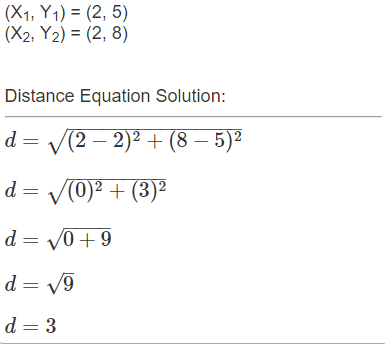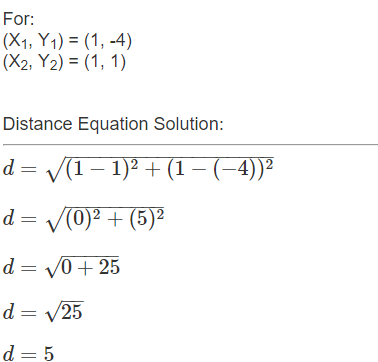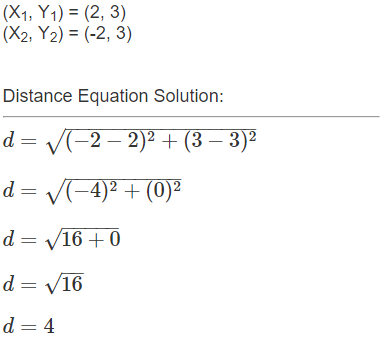Practice questions available in McGraw Hill Math Grade 6 Answer Key PDF Lesson 5.2 Distance will engage students and is a great way of informal assessment.

Exercises
Find The Distance Between The Points

Question 1.
(2, 5) and (2, 8) _________Explanation:
1. coordinates points = (2,5)
2. coordinates points = (2,8)
Distance between the two points = 3.

Question 2.
(-2, 1) and (-2, 3) _________Explanation:
1. coordinates points = (-2, 1)
2. coordinates points = (-2, 3)
Distance between the two points = 2.

Question 3.
(1, -4) and (1, 1) _________Explanation:
1. coordinates points = (1, -4)
2. coordinates points = (1, 1)
Distance between the two points = 5.

Question 4.
(3, 2) and (6, 2) _____Explanation:
1. coordinates points = (3, 2)
2. coordinates points = (6, 2)
Distance between the two points = 3.

Question 5.
(2, 3) and (-2, 3) _____Explanation:
1. coordinates points = (2,3)
2. coordinates points = (-2, 3)
Distance between the two points = 4.

Question 6.
(-4, -1) and (3, -1) _____